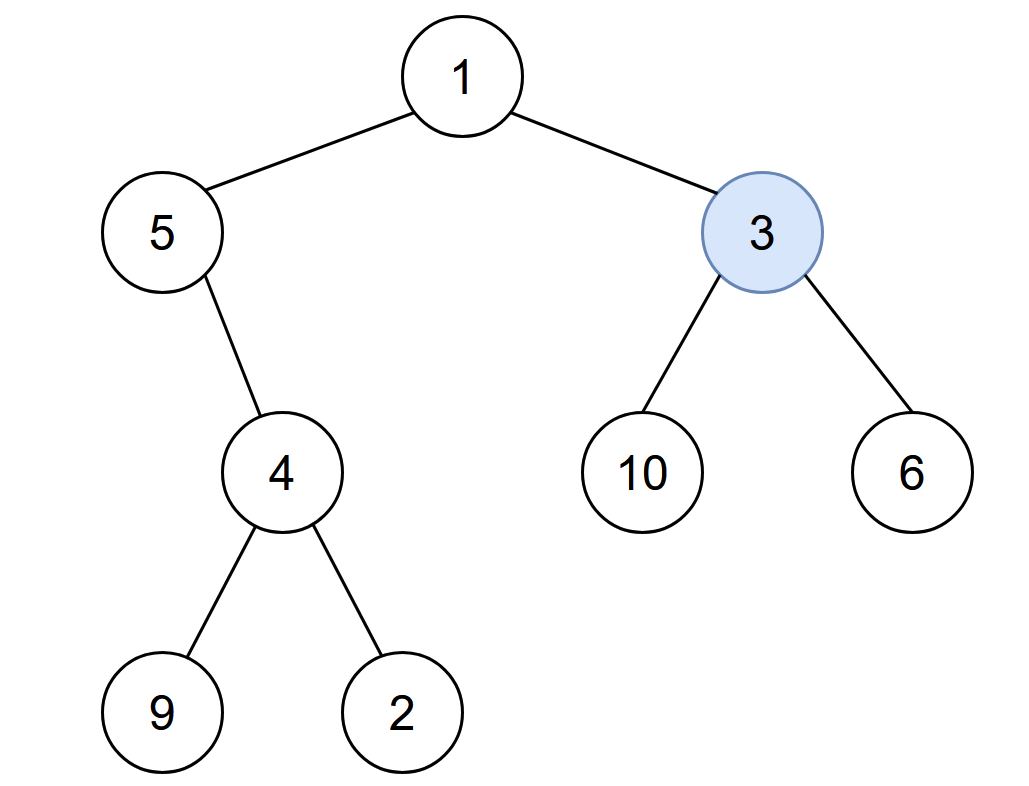2385. Amount of Time for Binary Tree to Be Infected
Medium
1.1K
13

You are given the `root` of a binary tree with unique values, and an integer `start`. At minute `0`, an infection starts from the node with value `start`.

Each minute, a node becomes infected if:

• The node is currently uninfected.
• The node is adjacent to an infected node.

Return the number of minutes needed for the entire tree to be infected.

Example 1:```Input: root = [1,5,3,null,4,10,6,9,2], start = 3
Output: 4
Explanation: The following nodes are infected during:
- Minute 0: Node 3
- Minute 1: Nodes 1, 10 and 6
- Minute 2: Node 5
- Minute 3: Node 4
- Minute 4: Nodes 9 and 2
It takes 4 minutes for the whole tree to be infected so we return 4.
```

Example 2:```Input: root = , start = 1
Output: 0
Explanation: At minute 0, the only node in the tree is infected so we return 0.
```

Constraints:

• The number of nodes in the tree is in the range `[1, 105]`.
• `1 <= Node.val <= 105`
• Each node has a unique value.
• A node with a value of `start` exists in the tree.
Accepted
25.1K
Submissions
44.2K
Acceptance Rate
56.9%

Seen this question in a real interview before?
1/4
Yes
No

Discussion (0)

Related Topics